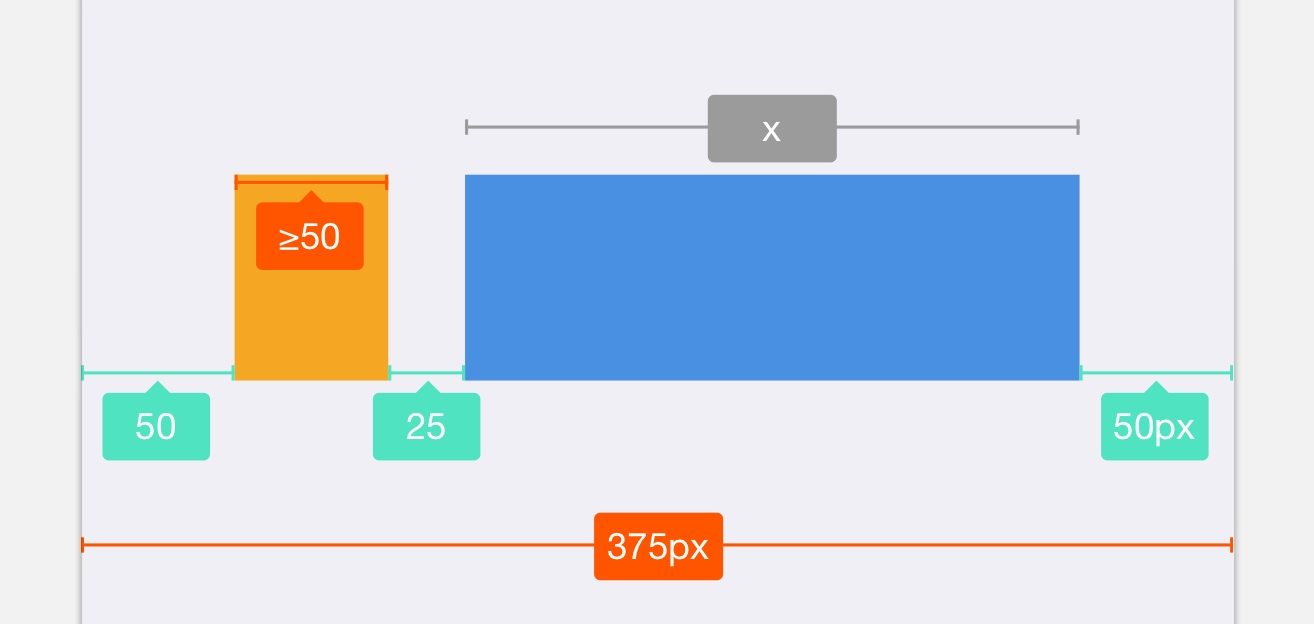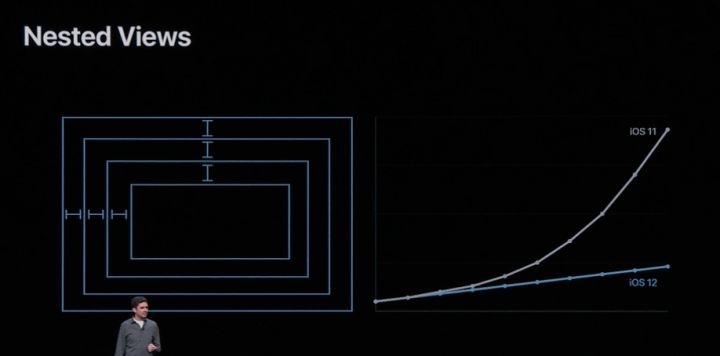desgard.com

温习线性规划Simplex 算法

Simplex 算法主要有三个步骤：

1. 找到一个初始的基本可行解；
2. 持续进行旋转（Pivot）操作；
3. 重复操作 2，直到找到最优解；

Simplex 算法我以下面一个线性规划方程为例：

Degeneracy can prevent the simplex algorithm from terminating, because it can lead to a phenomenon known as cycling: the slack forms at two different iterations of SIMPLEX are identical. Because of degeneracy, SIMPLEX could choose a sequence of pivot operations that leave the objective value unchanged but repeat a slack form within the sequence. Since SIMPLEX is a deterministic algorithm, if it cycles, then it will cycle through the same series of slack forms forever, never terminating.

1. 带入变量 $x_e$：目标条件中，系数为负数的第一个作为带入变量；
2. 替出变量 $x_i$：对所有约束条件中，选择对 $x_e$ 约束强度最大的一式；

Simplex 算法 Coding 及改善

• 矩阵 A：约束条件的系数矩阵；
• 矩阵 B：约束条件值矩阵；
• 矩阵 C：目标函数系数矩阵；

算法再修正

1. 若 $b_i$ 都为非负数。则直接使用原来的 Simplex 方法进行求解；
2. 否则引入 $x_0$，创建辅助线性规划 $L_aux$；
3. 松弛形式，选择 $b_i$ 最小项的那行带入，$x_0$ 作为替入变量进行一次旋转；
4. 求解 $L_aux$，若最优解为 0，说明有解。否则直接退出；
5. $x_0$ 为基本解，继续旋转，使其成为非基本变量；
6. 恢复原始目标函数，替换变量；
7. 使用原来的 Simplex 求解；

一个简单的场景复杂度分析

Simplex 算法再单次约束计算中是否十分高效呢，我们来讨论一下其复杂度。

1. 每次需要查找在目标方程中的一个负数 O(n)
2. 每次需要查找对应项方程 O(m)
3. 旋转操作中，每次需要执行 mat[i] = mat[i] - mat[row] * mat[i][col]。这个操作是对整个行进行操作，并且是按列下标遍历。np.arrange 操作每次会将行列扩展，我们将行定义为最大阈值 m+n，所以旋转开销 O(m*(m+n)) = O(mN)

总结